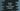# Linear search implementation in python## Write the linear search program in python:

Linear search is a search algorithm that searches for an item linearly, i.e. it starts from the leftmost element and keeps looking for that element one by one. If the element is found, it returns that.

In this post, we will learn how to use linear search to search for a number in an array using Python.

## Python program :

The below python program

``````def linearSearch(arr, num):
for i in range(len(arr)):
if arr[i] == num:
return i
return -1

given_arr = [1,4,55,32,11,33,21,22,45,88,99,101,98]
num_to_find = 45

print('{} is found at index :{}\n'.format(num_to_find, linearSearch(given_arr,num_to_find)))``````
• In this program, we have one function called linearSearch that takes one array arr and one number num to find using linear search.
• It uses one for loop to iterate through the elements of the array one by one.
• For each element, it checks if it is equal to the provided number num or not. If yes, it returns the value of i, i.e. the index of the number found.
• Else, it returns -1.

In the example, we are finding 45 in that array of numbers given_arr.

It will print the below output :

``45 is found at index :8``